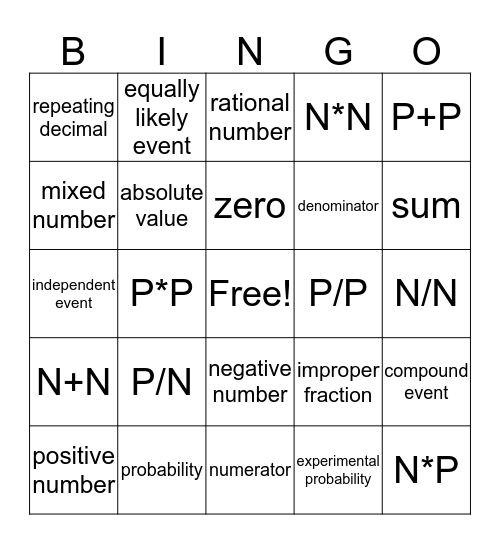# Math BINGOThis bingo card has a free space and 39 words: integer, opposite, additive inverse, absolute value, positive number, negative number, zero, zero pair, P+P, N+N, P*P, N*N, N*P, N/N, P/P, P/N, N/P, repeating decimal, terminating decimal, improper fraction, mixed number, numerator, denominator, sum, difference, product, quotient, likely event, unlikely event, equally likely event, certain, order of operations, rational number, probability, experimental probability, theoretical probability, compound event, independent event and dependent event.

## Play Online